Courses

# Mathematical Statistics - 2019 Past Year Paper

## 60 Questions MCQ Test IIT JAM Past Year Papers and Model Test Paper (All Branches) | Mathematical Statistics - 2019 Past Year Paper

Description
This mock test of Mathematical Statistics - 2019 Past Year Paper for IIT JAM helps you for every IIT JAM entrance exam. This contains 60 Multiple Choice Questions for IIT JAM Mathematical Statistics - 2019 Past Year Paper (mcq) to study with solutions a complete question bank. The solved questions answers in this Mathematical Statistics - 2019 Past Year Paper quiz give you a good mix of easy questions and tough questions. IIT JAM students definitely take this Mathematical Statistics - 2019 Past Year Paper exercise for a better result in the exam. You can find other Mathematical Statistics - 2019 Past Year Paper extra questions, long questions & short questions for IIT JAM on EduRev as well by searching above.
QUESTION: 1

Solution:
QUESTION: 2

Solution:
QUESTION: 3

### If F(x) =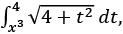for x ∈ ℝ, then F′(1) equals

Solution:
QUESTION: 4

Let T: ℝ2 → ℝ2 be a linear transformation such that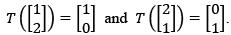Suppose that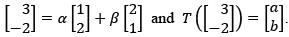Then α + β + a + b equals

Solution:
QUESTION: 5

Two biased coins C1 and C2 have probabilities of getting heads 2/3 and  3/4 , respectively, when tossed. If both coins are tossed independently two times each, then the probability of getting exactly two heads out of these four tosses is

Solution:
QUESTION: 6

Let X be a discrete random variable with the probability mass function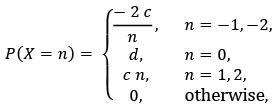where c and d are positive real numbers. If P(|X| ≤ 1) = 3/4, then E(X) equals

Solution:
QUESTION: 7

Let X be a Poisson random variable and P(X = 1) + 2 P(X = 0) = 12 P(X = 2). Which one of the following statements is TRUE?

Solution:
QUESTION: 8

Let X1, X2, … be a sequence of i.i.d. discrete random variables with the probability mass function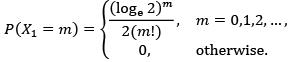If Sn = X1 + X2 + ⋯ + Xn , then which one of the following sequences of random variables converges to 0 in probability?

Solution:
QUESTION: 9

Let X1, X2, … , Xn be a random sample from a continuous distribution with the probability density function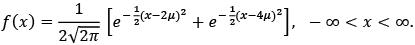If T = X1 + X2 + ⋯ + Xn , then which one of the following is an unbiased estimator of μ?

Solution:
QUESTION: 10

Let X1, X2, … , Xn be a random sample from a N(θ, 1) distribution. Instead of observing X1, X2, … , Xn, we observe Y1, Y2, … , Yn , where Yi = eXi , i = 1, 2, … , n. To test the hypothesis
H0: θ = 1 against H1: θ ≠ 1
based on the random sample Y1, Y2, … , Yn , the rejection region of the likelihood ratio test is of the form, for some c1 < c2 ,

Solution:
QUESTION: 11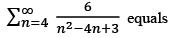Solution:
QUESTION: 12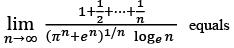Solution:
QUESTION: 13

A possible value of  b ∈ ℝ for which the equation  x4 + bx3 + 1 = 0 has no real root is

Solution:
QUESTION: 14

Let the Taylor polynomial of degree  20 for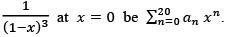Then a15 is

Solution:
QUESTION: 15

The length of the curve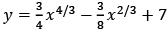from x = 1 to x = 8 equals

Solution:
QUESTION: 16

The volume of the solid generated by revolving the region bounded by the parabola x = 2y2 + 4 and the line x = 6 about the line x = 6 is

Solution:
QUESTION: 17

Let P be a 3 × 3 non-null real matrix. If there exist a 3 × 2 real matrix Q and a 2 × 3 real matrix R such that P = QR, then

Solution:
QUESTION: 18

If P =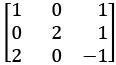and 6P−1 = aI3 + bP − P2, then the ordered pair (a, b) is

Solution:
QUESTION: 19

Let E, F and G be any three events with P(E) = 0.3, P(F|E) = 0.2, P(G|E) = 0.1 and P (F ∩ G|E) = 0.05. Then P(E − (F ∪ G)) equals

Solution:
QUESTION: 20

Let E and F be any two independent events with 0 < P(E) < 1 and 0 < P(F) < 1. Which one of the following statements is NOT TRUE?

Solution:
QUESTION: 21

Let X be a continuous random variable with the probability density function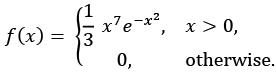Then the distribution of the random variable W = 2x2 is

Solution:
QUESTION: 22

Let X be a continuous random variable with the probability density function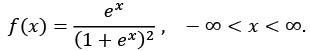Then E (X) and P(X > 1), respectively, are

Solution:
QUESTION: 23

The lifetime (in years) of bulbs is distributed as an Exp(1) random variable. Using Poisson approximation to the binomial distribution, the probability (round off to 2 decimal places) that out of the fifty randomly chosen bulbs at most one fails within one month equals

Solution:
QUESTION: 24

Let X follow a beta distribution with parameters m (> 0) and 2. If P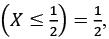then  Var(X) equals

Solution:
QUESTION: 25

Let X1, X2 and X3 be i.i.d. U(0,1) random variables. Then P(X1 > X2 + X3) equals

Solution:
QUESTION: 26

Let X and Y be i.i.d. U(0, 1) random variables. Then E(X| > Y) equals

Solution:
QUESTION: 27

Let −1 and 1 be the observed values of a random sample of size two from N(θ, θ) distribution. The maximum likelihood estimate of θ is

Solution:
QUESTION: 28

Let X1 and X2 be a random sample from a continuous distribution with the probability density function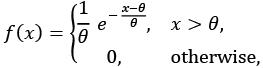where θ > 0. If X(1) = min{X1, X2} and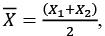then which one of the following statements is TRUE?

Solution:
QUESTION: 29

Let X1, X2, … , Xn be a random sample from a continuous distribution with the probability density function f(x). To test the hypothesis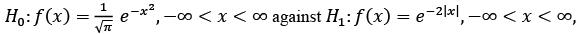the rejection region of the most powerful size α test is of the form, for some c > 0,

Solution:
QUESTION: 30

Let X1, X2, … , Xn be a random sample from a N(θ, 1) distribution. To test H0: θ = 0 against H1: θ = 1, assume that the critical region is given by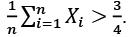Then the minimum sample size required so that P(Type I error) ≤ 0.05 is

Solution:
*Multiple options can be correct
QUESTION: 31

Let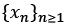be a sequence of positive real numbers such that the series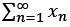converges. Which of the following statements is (are) always TRUE?

Solution:
*Multiple options can be correct
QUESTION: 32

Let f : ℝ → ℝ be continuous on ℝ and differentiable on  (−∞, 0) ∪ (0, ∞). Which of the following statements is (are) always TRUE?

Solution:
*Multiple options can be correct
QUESTION: 33

Let P be a  2 × 2 real matrix such that every non-zero vector in  ℝ2 is an eigenvector of P. Suppose that  λ1 and  λ2 denote the eigenvalues of  P and  P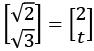for some  t ∈ ℝ. Which of the following statements is (are) TRUE?

Solution:
*Multiple options can be correct
QUESTION: 34

Let P be an n x n non-null real skew-symmetric matrix, where n is even. Which of the following statements is (are) always TRUE?

Solution:
*Multiple options can be correct
QUESTION: 35

Let X be a random variable with the cumulative distribution function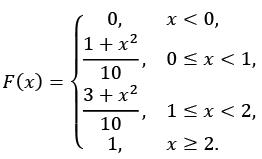Which of the following statements is (are) TRUE?

Solution:
*Multiple options can be correct
QUESTION: 36

Let X and Y be i.i.d. Exp (λ) random variables. If Z = max{X − Y, 0}, then which of the following statements is (are) TRUE?

Solution:
*Multiple options can be correct
QUESTION: 37

Let the discrete random variables X and Y have the joint probability mass function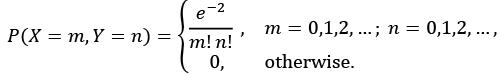Which of the following statements is (are) TRUE?

Solution:
*Multiple options can be correct
QUESTION: 38

Let X1, X2, … be a sequence of i.i.d. continuous random variables with the probability density function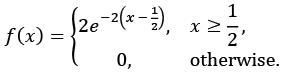If Sn = X1 + X2 + ⋯ + Xn and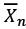= s/n, then the distributions of which of the following sequences of random variables converge(s) to a normal distribution with mean 0 and a finite variance?

Solution:
*Multiple options can be correct
QUESTION: 39

Let X1, X2, … , Xn be a random sample from a U(θ, 0) distribution, where θ < 0.
If Tn = min{X1, X2, … , Xn }, then which of the following sequences of estimators is (are) consistent for θ?

Solution:
*Multiple options can be correct
QUESTION: 40

Let X1, X2, … ,  be a random sample from a continuous distribution with the probability density function, for  λ > 0,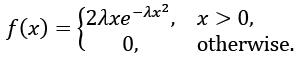To test the hypothesis H0: λ = 1/2 against H1: λ = 3/4 at the level α (0 < α < 1), which of  the following statements is (are) TRUE?

Solution:
*Answer can only contain numeric values
QUESTION: 41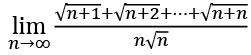(round off to 2 decimal places) equals __________

Solution:
*Answer can only contain numeric values
QUESTION: 42

Let f : [0, 2] → ℝ be such that |f(x) − f(y)| ≤ |x − y|4/3 for all x, y ∈ [0, 2].
If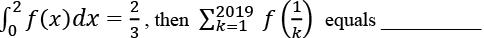Solution:
*Answer can only contain numeric values
QUESTION: 43

The value (round off to 2 decimal places) of the double integral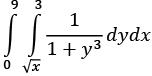equals __________

Solution:
*Answer can only contain numeric values
QUESTION: 44

If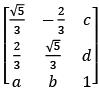is a real orthogonal matrix, then a2 + b2 + c2 + d2 equals ________

Solution:
*Answer can only contain numeric values
QUESTION: 45

Two fair dice are tossed independently and it is found that one face is odd and the other one is even. Then the probability (round off to 2 decimal places) that the sum is less than 6 equals __________

Solution:
*Answer can only contain numeric values
QUESTION: 46

Let X be a random variable with the moment generating function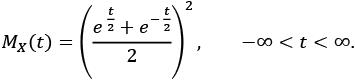Using Chebyshev’s inequality, the upper bound for P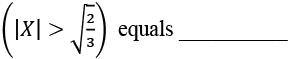Solution:
*Answer can only contain numeric values
QUESTION: 47

In a production line of a factory, each packet contains four items. Past record shows that 20% of the produced items are defective. A quality manager inspects each item in a packet and approves the packet for shipment if at most one item in the packet is found to be defective. Then the probability (round off to 2 decimal places) that out of the three randomly inspected packets at least two are approved for shipment equals __________

Solution:
*Answer can only contain numeric values
QUESTION: 48

Let X be the number of heads obtained in a sequence of 10 independent tosses of a fair coin. The fair coin is tossed again X number of times independently, and let Y be the number of heads obtained in these X number of tosses. Then E(X + 2Y) equals __________

Solution:
*Answer can only contain numeric values
QUESTION: 49

Let 0, 1, 0, 0, 1 be the observed values of a random sample of size five from a discrete distribution with the probability mass function P(X = 1) = 1 − P(X = 0) = 1 − e −λ , where λ > 0. The method of moments estimate (round off to 2 decimal places) of λ equals __________

Solution:
*Answer can only contain numeric values
QUESTION: 50

Let X1, X2, X3 be a random sample from N(μ1, σ2 ) distribution and Y1, Y2, Y3 be a random sample from N(μ2, σ2) distribution. Also, assume that (X1, X2, X3) and (Y1, Y2, Y3) are independent. Let the observed values of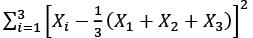and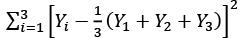be 1 and 5, respectively. The length (round off to 2 decimal places) of the shortest 90% confidence interval of μ1 − μ2 equals __________

Solution:
*Answer can only contain numeric values
QUESTION: 51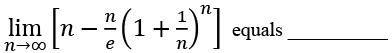Solution:
*Answer can only contain numeric values
QUESTION: 52

For any real number y, let [y] be the greatest integer less than or equal to y and let {y} = y − [y]. For n = 1, 2, …, and for  x ∈ ℝ, let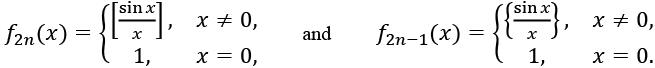Then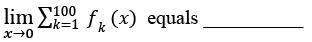Solution:
*Answer can only contain numeric values
QUESTION: 53

The volume (round off to 2 decimal places) of the region in the first octant (x ≥ 0, y ≥ 0, z ≥ 0 ) bounded by the cylinder x2 + x2 = 4 and the planes z = 2 and y + z = 4 equals __________

Solution:
*Answer can only contain numeric values
QUESTION: 54

If ad − bc = 2 and ps − qr = 1, then the determinant of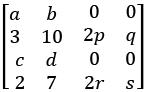equals _________.

Solution:
*Answer can only contain numeric values
QUESTION: 55

In an ethnic group, 30% of the adult male population is known to have heart disease. A test indicates high cholesterol level in 80% of adult males with heart disease. But the test also indicates high cholesterol levels in 10% of the adult males with no heart disease. Then the probability (round off to 2 decimal places), that a randomly selected adult male from this population does not have heart disease given that the test indicates high cholesterol level, equals __________

Solution:
*Answer can only contain numeric values
QUESTION: 56

Let X be a continuous random variable with the probability density function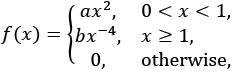where a and b are positive real numbers. If E (X) = 1, then E(X2) equals __________

Solution:
*Answer can only contain numeric values
QUESTION: 57

Let X and Y be jointly distributed continuous random variables, where Y is positive valued with  E(Y2) = 6. If the conditional distribution of X given Y = y is y(1 − y, 1 + y), then Var(X) equals __________

Solution:
*Answer can only contain numeric values
QUESTION: 58

Let X1, X2, … , X10 be i.i.d. N(0, 1) random variables. If T =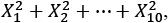, then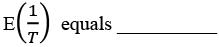Solution:
*Answer can only contain numeric values
QUESTION: 59

Let X1, X2, X3 be a random sample from a continuous distribution with the probability density function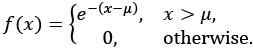Let X(1) = min{X1, X2, X3} and c > 0 be a real number. Then (X(1) − c, X(1)) is a 97% confidence interval for μ, if c (round off to 2-decimal places) equals __________.

Solution:
*Answer can only contain numeric values
QUESTION: 60

Let x1, X2, X3, X4 be a random sample from a discrete distribution with the probability mass function P(X = 0) = 1 − P( = 1) = 1 − p, for 0 < p < 1. To test the hypothesis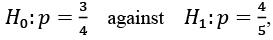consider the test:
Reject H0 if X1 + X2 + X3 + X4 > 3.
Let the size and power of the test be denoted by α and y, respectively. Then α + y(round off to 2 decimal places) equals __________.

Solution:

Track your progress, build streaks, highlight & save important lessons and more!

### Similar Content### Related tests